• 一、实验目的(1)综合应用信号频谱分析和数字滤波器设计的知识，实现信号的滤波。(2)比较分析干扰信号加入前、后的频谱有何区别，...二、实验原理(1) FIR数字滤波器的设计原理：FIR数字滤波器总是稳定的系统，且可以...
一、实验目的(1)综合应用信号频谱分析和数字滤波器设计的知识，实现信号的滤波。(2)比较分析干扰信号加入前、后的频谱有何区别，分析噪声的影响。(3)加深理解信号时域和频域分析的物理概念，认识不同滤波器的特性和适用范围。(4)掌握设计IIR、FIR 数字滤波器的原理和特性，通过比较对两种滤波器的滤波作用有直观认识。二、实验原理(1) FIR数字滤波器的设计原理：FIR数字滤波器总是稳定的系统，且可以设计成具有线性相位。M阶FIR数字滤波器的系统函数为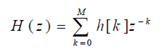系统的单位脉冲响应h[k]是长度为M+1的有限长因果序列。当满足h[k] =±h[M -k]的对称条件时，该FIR数字滤波器具有线性相位。FIR数字滤波器设计方法主要有窗口法、频率取样法、优化设计法。MATLAB 中常用FIR数字滤波器设计函数： fir1 窗函数法FIR数字滤波器设计：低通、高通、带通、带阻、多频带滤波器。 fir2 频率抽样法FIR数字滤波器设计：任意频率响应。 firls FIR数字滤波器设计：指定频率响应。(2) IIR 数字滤波器的设计原理：IIR数字滤波器一般为线性时不变的因果离散系统，N阶IIR数字滤波器的系统函数可以表达为z-1的有理多项式，即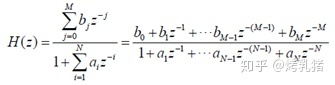分母系数中{；i=1,2,…,N}至少有一个非零。对于因果IIR数字滤波器，满足M≤ N。IIR数字滤波器的设计主要通过成熟的模拟滤波器设计方法来实现。首先在频域将数字滤波器设计指标转化为模拟低通滤波器设计指标，将任意模拟滤波器转换为原型模拟低通滤波器设计指标，根据模拟低通滤波器设计指标设计出模拟低通滤波器Hlp(s)，由Hlp(s)经过相应的复频域变换得到H(s)，由H(s)经过脉冲响应不变法或双线性变换法得到所需的IIR数字滤波器H(z)，由此可见，IIR数字滤波器的设计的重要环节是模拟低通滤波器的设计，涉及低通模拟滤波器的方法有巴特沃斯，切比雪夫和椭圆等滤波器设计方法。用 MATLAB 设计IIR滤波器的常用函数： IIR滤波器阶数选择： Buttord-------巴特沃斯（Butterworth）滤波器阶数选择。 Cheblord-----切比雪夫（Chebyshev）I 型滤波器阶数选择。 Cheb2ord----切比雪夫（Chebyshev）II 型滤波器阶数选择。 Ellpord-------椭圆（Elliptic）滤波器阶数选择。 IIR滤波器设计： Butter-------巴特沃斯（Butterworth）滤波器设计。 cheby1------切比雪夫（Chebyshev）I 型滤波器设计。 cheby2------切比雪夫（Chebyshev）II 型滤波器设计。 maxflat------通用的巴特沃斯（Butterworth）低通滤波器设计。三、实验步骤1利用 wavread 函数读入一段音乐信号(.wav)，利用 FFT 分析该信号的频谱。[y,FS]=audioread('C:UsersDesktopwind.mp3');
music=y(:,1);   %由于原MP3音频为双音轨，除去一条
N=length(music);    %获取原音频的采样点数
w=linspace(0,2*pi,N);   %设置数字角频率范围
MUSIC=fft(music);   %求原音乐信号频谱
subplot(2,2,1)
pMUSIC=abs(MUSIC);  %绘制原信号幅度谱
plot(w,pMUSIC);
title('原始音乐频谱');
ylabel('幅度模值');
audiowrite('wind1.wav',music,FS);  %保存除去一条音轨的文件
T=1/FS; %开始描述噪声信号
w1=2*pi*500*T;  %将模拟角频率转化为数字角频率
w2=2*pi*1000*T;
for n=1:N
noise(n)=(sin(w1*n)+sin(w2*n))*0.1; %调整合适噪声大小，不要把原信号全部淹没
end
NoiseMusic=music+noise';    %将噪声加入原信号
subplot(2,2,2)
pnoise=abs(fft(noise)); %绘制噪声频谱
plot(w,pnoise);    %加abs画幅度谱
title('噪声频谱');
ylabel('幅度模值');
subplot(2,2,3)
pNoiseMusic=abs(fft(NoiseMusic));
plot(w,pNoiseMusic);
title('加入噪声后音乐频谱');
ylabel('幅度模值');
audiowrite('windnoise.wav',NoiseMusic,FS);  %保存加入噪声的音频
Rp=2;   %设置通带波纹为2dB
Rs=60;  %设置阻带衰减为60dB
%除去500Hz干扰信号
wp=[20,700]*2/FS;   %设置通带区间
ws=[499,501]*2/FS;  %设置阻带区间
[n,wn]=buttord(wp,ws,Rp,Rs);    %巴特沃斯滤波器阶数选择
[b,a]=butter(n,wn,'stop');  %设计带阻巴特沃斯滤波器
music1=filter(b,a,NoiseMusic); %求经过除去500Hz带阻后的信号
%除去1000Hz干扰信号
wp1=[900,1300]*2/FS;
ws1=[999,1001]*2/FS;
[n,wn]=buttord(wp1,ws1,Rp,Rs);
[b1,a1]=butter(n,wn,'stop');
music2=filter(b1,a1,music1);
%绘制除去500Hz和1000Hz干扰信号后的音乐频谱
subplot(2,2,4)
pmusic2=abs(fft(music2));
plot(w,pmusic2);
title('除去噪声后的频谱');
ylabel('幅度模值');
audiowrite('windpure.wav',NoiseMusic,FS);   %保存除噪声后的信号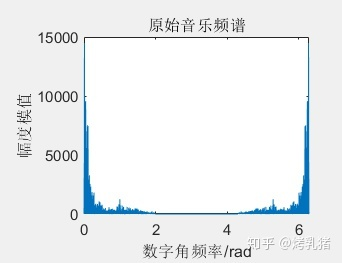2在该段音乐信号中加入两个频率分别为 500Hz 和 1000Hz 的正弦型干扰信号，利用 FFT 观察该信号的频谱。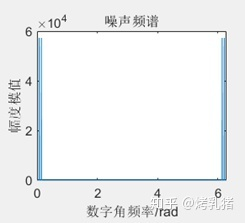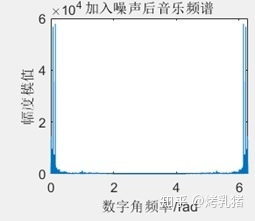3根据其频谱，设计不同类型的滤波器（IIR，FIR）滤除干扰信号，从时域（通过播放）和频域（通过频谱）比较结果。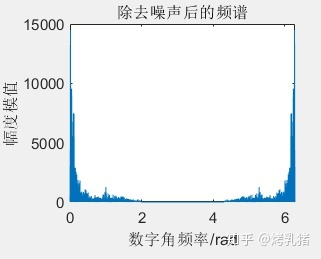通过播放之后发现滤波效果是非常不错的，噪声已经基本被滤去。上为两个文件，一个为滤波之前加入噪声的音乐文件windnoise.wav,后者为滤波之后的windpure.wav.四、思考题1.利用频率选择滤波器（FIR 滤波器、IIR 滤波器）进行信号去噪的基本思想及主要步骤是什么？ 基本思想：利用频率选择滤波器的传输选择特性，对输入信号进行加工和变换，改变输入序列的频谱或信号波形，使需要的频率分量通过，抑制无用的信号分量。主要步骤：首先根据输入信号的频谱特性及设计目标要求确定需要使用的滤波器类型，包括低通、高通、带通、带阻。其次，由需要的相位特性及幅度特性确定滤波器的设计方法，包括FIR（巴特沃斯、切比雪夫）、IIR(巴特沃斯、切比雪夫)，然后根据目标设计要求确定滤波器参数，需要的参数根据滤波器的设计方法确定，主要包括滤波器的阶次、频率要求、幅度响应要求，之后再利用设计好的滤波器对实际信号进行处理，并检验是否满足设计要求，并调整和优化。2.如何根据含有噪声信号的频谱特性选择滤波器的类型和设计指标？ 根据采集到的信号获得频谱图，由时域频域对应关系，确定需要滤除噪声的特性，然后从以下角度确定所需要的滤波器：首先是频响特性，IIR滤波器设计时不考虑相位特性，且通常相位都是非线性的，而FIR滤波器在满足幅频特性要求的同时，还能获得比较严格的线性相位特性，利用窗函数或者其他算法可以逼近更加任意的频响特性，因此性能更优越，适用范围更广。其次是稳定性问题，IIR滤波器设计时，极点必须在单位圆之内，而FIR滤波器极点在单位圆内，因此始终稳定。然后考虑滤波器的结构，IIR滤波器一般采用递归结构，存在有输出对输入的反，而IIR滤波器的阶次相对较低，运算次数少，存储单元少，FIR滤波器正好相反。最后考虑设计工作量，FIR无表可查，需要用到迭代法，计算量较大，而IIR滤波器相对简单，有现成的计算公式和数据表格可以查阅。实验二 图像滤波实验一、实验目的设计一个9696像素的离散空间图像。假定图像为一个棋盘，由8*8的交替变化的黑白方块组成，如图。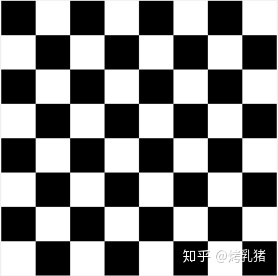（a） 用冲激响应为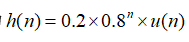的滤波器对图像进行逐行滤波，然后逐列滤波。用matlab的imagesc命令在屏幕上显示图像。（b） 用冲激响应为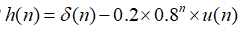的滤波器对图像进行滤波，然后进行逐列滤波。用matlab的imagesc命令在屏幕上显示图像。二、实验结果N=96;   %设置分辨率
p=ones(N,N);
for m=1:96  %将题目中所述图像二值化
if mod(floor((m-1)/12),2)==0
for n=1:96
if mod(floor((n-1)/12),2)==0
p(m,n)=0;
end
end
else
for n=1:96
if mod(floor((n-1)/12),2)==1
p(m,n)=0;
end
end
end
end
colormap gray
subplot(1,3,1)
imshow(p);  %绘制原图像
title('原图像');
for n=1:96
h1(n)=0.2*0.8^n;    %求(a)中的冲激响应
end
h2(1)=1-0.2*0.8;    %求(b)中的冲激响应
for n=2:96
h2(n)=-0.2*0.8^n;
end
%接下来求变化后图像
for i=1:96
p1(i,:)=ifft(fft(p(i,:)).*fft(h1)); %求经过h1逐行滤波后的图像
p2(i,:)=ifft(fft(p(i,:)).*fft(h2)); %求经过h2逐行滤波后的图像
end
h11=h1';
h21=h2';
for i=1:96
p1(:,i)=ifft(fft(p1(:,i)).*fft(h11)); %求经过h1逐列滤波后的图像
p2(:,i)=ifft(fft(p2(:,i)).*fft(h21)); %求经过h2逐列滤波后的图像
end
subplot(1,3,2)
imshow(p1);
title('经过(a)所示滤波器图样');
subplot(1,3,3)
imshow(p2);
title('经过(b)所示滤波器图样');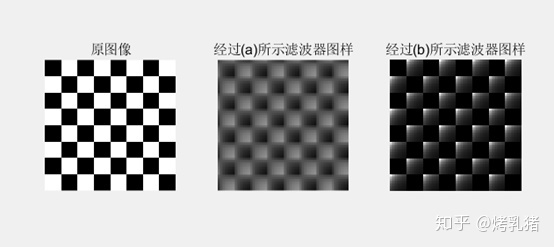展开全文• FIR设计-基于MatlabFIR低通滤波器的设计与仿真.pdf 基于MatlabFIR低通滤波器的设计与仿真.pdf FIR设计 FIR滤波器设计与仿真。
• 使用MATLAB设计FIR低通滤波器 ** 关于现代通信原理作业。 https://blog.csdn.net/tanghonghanhaoli/article/details/100533581 这是老师给出的提示，里面有比较详细的讲解，下面写一些我的想法。 1. 为什么要设计低...
**
使用MATLAB设计FIR低通滤波器
**
关于现代通信原理作业。
https://blog.csdn.net/tanghonghanhaoli/article/details/100533581
这是老师给出的提示，里面有比较详细的讲解，下面写一些我的想法。
1. 为什么要设计低通滤波器
我们可以看到例子中给的m(t)在时域中已经是物理可实现的信号了，但是在频域上看这个信号的频谱是无限宽的，显然这用计算机是难以处理的，加上Sa信号旁瓣的影响并不是很大，所以我们考虑滤除旁瓣，即使用低通滤波器。
2. 关于如何设计低通滤波器的理论分析
对于例题中是想保留Sa信号±10Hz内的频率分量，即需要设计一个频率范围在±10Hz的低通滤波器h(t)，也就是频域为一个方波信号H(f)。由信号与系统的知识可得，频域方波，时域则为Sa信号。时域h(t)如果为标准的Sa函数，则在t<0的时候，h(t)还会有值，这明显是物理不可实现的，所以这里考虑将h(t)进行一定的时移。
注意：这里并不会影响滤波器的功能，因为时域平移对应频域的相移，但是幅度没有改变，所以即使时域平移了，频域的幅频特性并不会改变，依然是在±10Hz通过信号。
3. 关于MATLAB的Filter Designer
在MATLAB的Filter Designer中就将中心移到了(N/2)*Ts，N就是对h(t)的抽样点个数。时域抽样点越多，对应的时域波形就越接近Sa信号，频域就越接近方波，即越接近我们要的低通滤波器。同时，如果抽样频率Fs不变，则Ts也不会改变，抽样点N增加，那么中心平移得就更多了，即时延就更多了；换个角度理解，抽样点更多，抽样时间间隔Ts不变，也就意味着对更高频的旁瓣也会被采样，则被采样的Sa信号更宽了，占用的时间也更多了，也能想象时延会更大。
但是在MATLAB中，N好像是由系统根据你设置的参数自动匹配的。我没有看到设置抽样个数N的选项，只有设置抽样频率Fs的选项，N会根据你设置的Fs进行自动匹配，就是设置完参数后点击Design Filter后，左上角看到的order的数值。Fs增加，即Ts减小，N会随之增加，在MATLAB中，我们可以看到两者的变化速率是一致的，所以中心始终在一个位置。（所以我在想是不是MATLAB关于Design Filter的系统设置就是只采样主瓣和相邻几个旁瓣的数值，这样也确实已经很接近一个Sa信号了，更高频的旁瓣其实幅度很小，近似为0应该也不会带来太多误差）本人学生，难免学艺不精，若有疏漏错误之处，敬请指正!


展开全文• 本程序是用matlab实现的FIR低通滤波器，通过加载一段音频文件，进行滤波处理，最后又回放滤波器后的音频文件…… 可以通过听觉上区分滤波前后的差别，更好的方式当然是通过观察滤波前后的频谱来进行验证……
• 基于MATLAB的设计fir低通滤波器，采用方法是频率抽样法
• 基于MATLAB做的FIR低通滤波器，newfft是自己编写的，也可以直接调用MATLAB内部的fft程序，runfir文件作为一个运行的实例，数字信号处理方面可能用得到。
• 基于FPGA低通滤波器FIR的设计1 滤波器的特征参数介绍图1 低通滤波器特征参数 如图1所示，低通滤波器的通带截止频率为ωp ,通带容限为α1，阻带截止频率为ωs,阻带容限为α2。通带定义为|ω|≤ωp ,过渡带定义为ωp2 ...


基于FPGA低通滤波器FIR的设计1 滤波器的特征参数介绍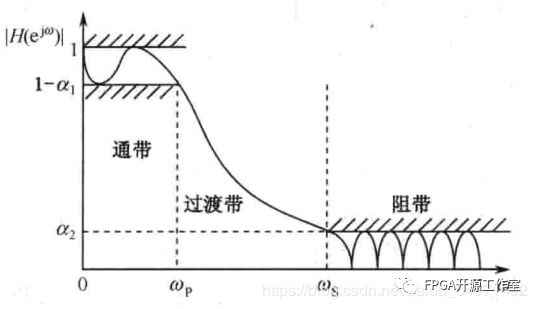图1 低通滤波器特征参数       如图1所示，低通滤波器的通带截止频率为ωp ,通带容限为α1，阻带截止频率为ωs,阻带容限为α2。通带定义为|ω|≤ωp ,过渡带定义为ωp2 设计目标      要求：使用fir滤波器设计一个滤波器系数为15阶的低通滤波器，通带截止频率为1KHZ，采样频率为44.1khz。3 matlab的设计验证close allclear allclc%设计一个低通滤波器 采样频率为44.1khz, 截止频率为1khzFS = 44100; %HZfc = 1000;N  = 15;Q  = 16;%以采样频率的一半，对频率进行归一化处理wn_lpf=fc*2/FS;%采用fir1函数设计FIR滤波器b_lpf=fir1(N-1,wn_lpf);%滤波系数进行量化b_16=round(b_lpf/max(abs(b_lpf))*(2^(Q-1)-1));%求滤波器的幅频响应m_lpf=20*log(abs(fft(b_lpf)))/log(10);b16_lpf=20*log(abs(fft(b_16)))/log(10);%设置幅频响应的横从标单位为Hzx_f=[0:(FS/length(m_lpf)):FS/2];  %绘制单位脉冲响应subplot(221);stem(b_lpf);xlabel('n');ylabel('h(n)');title('低通滤波器的单位脉冲响应','fontsize',8);subplot(222);stem(b_16);xlabel('n');ylabel('h(n)');title('低通滤波器的单位脉冲响应','fontsize',8);%绘制幅频响应曲线subplot(223);plot(x_f,m_lpf(1:length(x_f)));xlabel('频率(Hz)','fontsize',8);ylabel('幅度(dB)','fontsize',8);title('低通滤波器的幅频响应','fontsize',8);subplot(224);plot(x_f,b16_lpf(1:length(x_f)));xlabel('频率(Hz)','fontsize',8);ylabel('幅度(dB)','fontsize',8);title('低通滤波器的幅频响应','fontsize',8);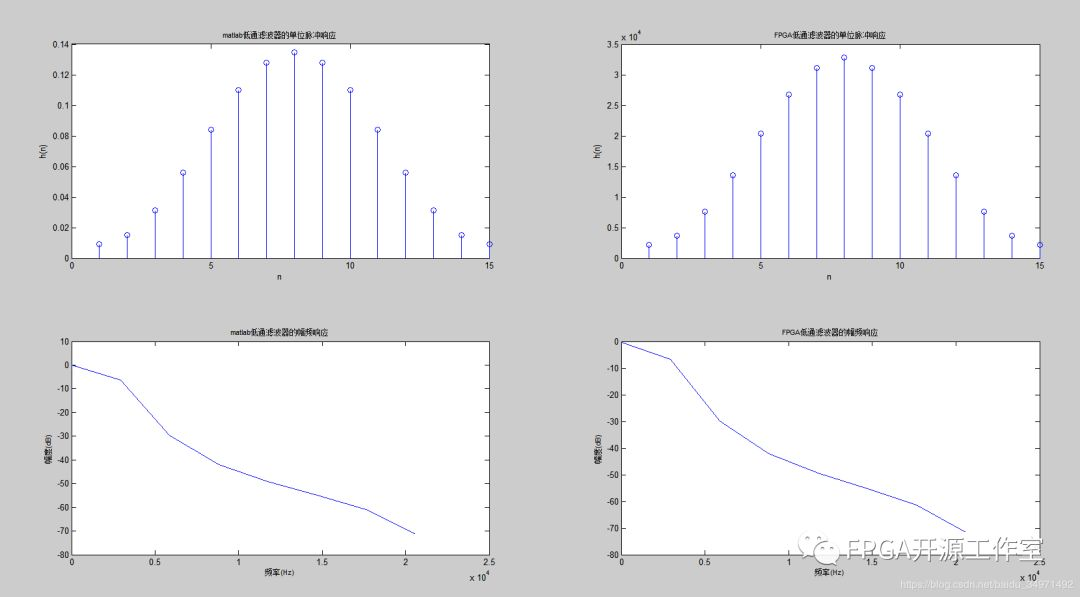图2 低通滤波器的matlab单位脉冲响应和幅频响应4 FPGA信号发生器为了验证低通滤波器，我们设计了测试验证的sin信号发生器。wire [17:0] kfreq= (k<2048)?18’h00333:18’h00FFF;//1khz-5khz产生1khz和5khz的sin波。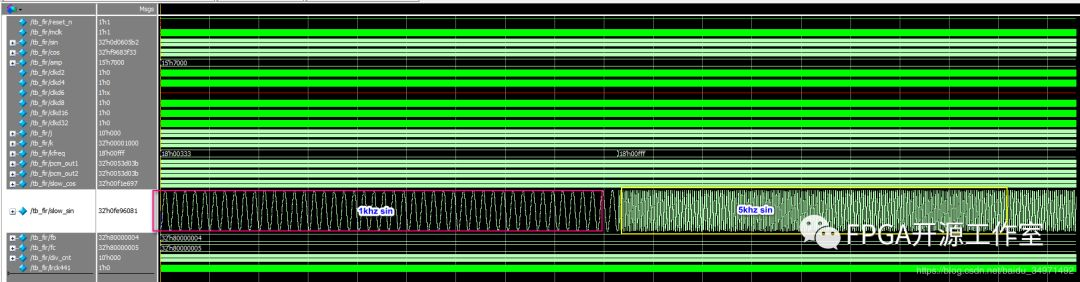图3 信号发生器产生1khz和5khz的sin测试源测试源的FFT分析：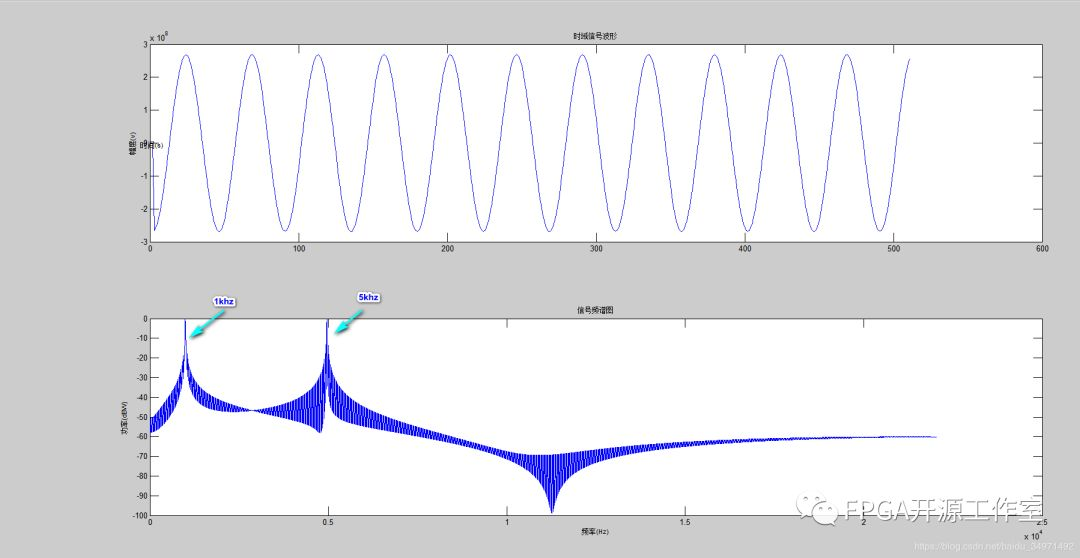图4 测试源数据的matlab分析      由图4的频域图可知，测试源产生了1khz和5khz的sin。5 FPGA的fir设计      Fir滤波器verilog代码设计采取全并行模式。timescale 1ps/1psmodule fir1(           input mclk,//45.1584MHZ	   input reset_n,	   input signed[31:0] pcm_in,	   output signed[31:0] pcm_out);localparam LAST_CYCLE = 1023;reg [9:0] i;reg signed [31:0] pcm1,pcm2,pcm3,pcm4,pcm5,pcm6,pcm7,pcm8,pcm9,pcm10,pcm11,pcm12,pcm13,pcm14,pcm15;reg signed [47:0] pcm1_out,pcm2_out,pcm3_out,pcm4_out,pcm5_out,pcm6_out,pcm7_out,pcm8_out,pcm9_out,pcm10_out,pcm11_out,pcm12_out,pcm13_out,pcm14_out,pcm15_out;reg signed [51:0] pcm_r;wire signed [15:0] coeff1,coeff2,coeff3,coeff4,coeff5,coeff6,coeff7,coeff8,coeff9,coeff10,coeff11,coeff12,coeff13,coeff14,coeff15;assign coeff1 = 2208;assign coeff2 = 3631;assign coeff3 = 7612;assign coeff4 = 13576;assign coeff5 = 20413;assign coeff6 = 26727;assign coeff7 = 31169;assign coeff8 = 32767;assign coeff9 = 31169;assign coeff10 = 26727;assign coeff11 = 20413;assign coeff12 = 13576;assign coeff13 = 7612;assign coeff14 = 3631;assign coeff15 = 2208;assign pcm_out = pcm_r[51:20];always @(posedge mclk or negedge reset_n) beginif(!reset_n) begin    i<= 0;	pcm1<=0;	pcm2<=0;	pcm3<=0;	pcm4<=0;	pcm5<=0;	pcm6<=0;	pcm7<=0;	pcm8<=0;	pcm9<=0;	pcm10<=0;	pcm11<=0;	pcm12<=0;	pcm13<=0;	pcm14<=0;	pcm15<=0;    pcm1_out<=0;	pcm2_out<=0;	pcm3_out<=0;	pcm4_out<=0;	pcm5_out<=0;	pcm6_out<=0;	pcm7_out<=0;	pcm8_out<=0;	pcm9_out<=0;	pcm10_out<=0;	pcm11_out<=0;	pcm12_out<=0;	pcm13_out<=0;	pcm14_out<=0;	pcm15_out<=0;	pcm_r<= 0;  endelse begin    i <= i + 1;if(i == 0) begin	  pcm1<=pcm_in;	  pcm2<=pcm1;	  pcm3<=pcm2;	  pcm4<=pcm3;	  pcm5<=pcm4;	  pcm6<=pcm5;	  pcm7<=pcm6;	  pcm8<=pcm7;	  pcm9<=pcm8;	  pcm10<=pcm9;	  pcm11<=pcm10;	  pcm12<=pcm11;	  pcm13<=pcm12;	  pcm14<=pcm13;	  pcm15<=pcm14;	endif(i==1) begin    	pcm1_out <= pcm1*coeff1;	    pcm2_out <= pcm2*coeff2;	    pcm3_out <= pcm3*coeff3;	    pcm4_out <= pcm4*coeff4;	    pcm5_out <= pcm5*coeff5;	    pcm6_out <= pcm6*coeff6;	    pcm7_out <= pcm7*coeff7;	    pcm8_out <= pcm8*coeff8;	    pcm9_out <= pcm9*coeff9;	    pcm10_out <= pcm10*coeff10;	    pcm11_out <= pcm11*coeff11;	    pcm12_out <= pcm12*coeff12;	    pcm13_out <= pcm13*coeff13;	    pcm14_out <= pcm14*coeff14;	    pcm15_out <= pcm15*coeff15;	endif(i==2) pcm_r<= pcm1_out +pcm2_out+pcm3_out+pcm4_out+pcm5_out+pcm6_out+pcm7_out+pcm8_out+pcm9_out+pcm10_out+pcm11_out+pcm12_out+pcm13_out+pcm14_out+pcm15_out;  endendendmodule     fpga实验modelsim仿真结果，从图5来看1khz几乎保持不变，而5khz波形幅度上被抑制了很多。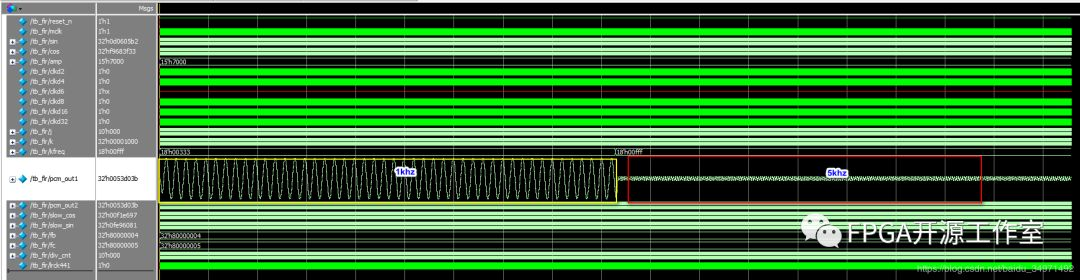图5 modelsim fir实验结果时域波形Matlab fft分析：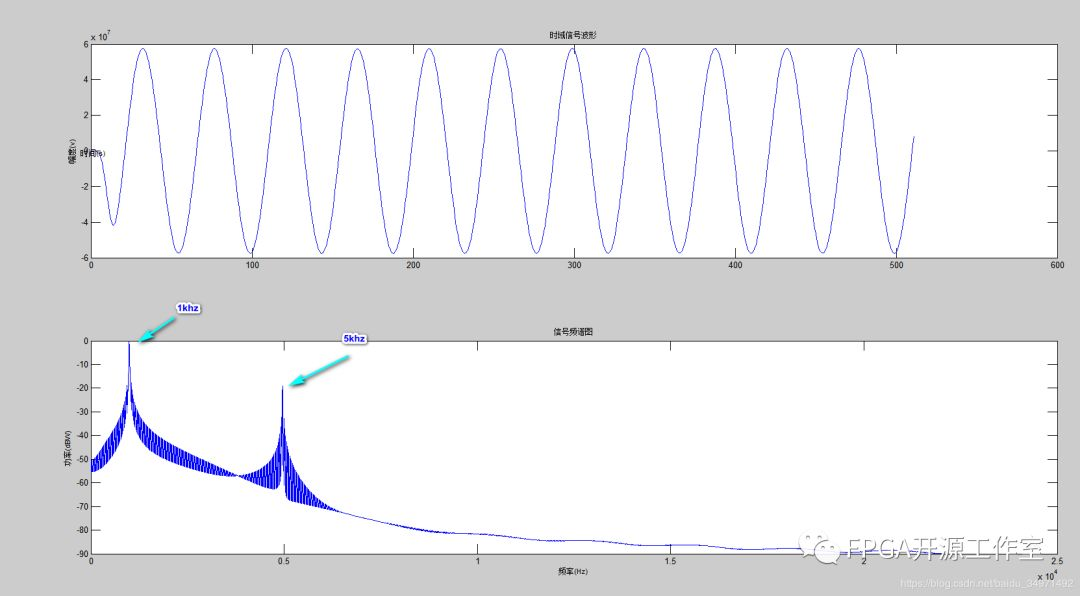图6 matlab分析实验结果      由图2和图6对比，5khz经过滤波后的功率减小了20DB，图2与图5基本一致，fir的低通滤波器全并行设计成功。DSP往期音频总线I2S协议信号的产生信号的基本概念基于LUT的DDS的设计更多精彩推荐，请关注我们万水千山总是情，点个 “好看” 行不行！！！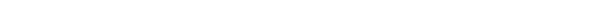展开全文• 简述用MATLAB设计低通滤波器的程序，主要针对MATLAB得的初学者!
• matlab实现单声道立体声信号的fir低通滤波，使用窗函数法，并将输入数据进行输出，供硬件仿真使用。
• 3 2007 年 5 月 Journal of Kashgar Teachers College May 2007 基于 MATLABFIR数字低通滤波器 分析和设计Ξ雷学堂 ,方志雄 (黄冈师范学院 物理科学与技术系 ,湖北 黄冈 438000) 摘 要 :以 FIR 数字低通滤波器为...
第 28 卷第 3 期 喀什师范学院学报 Vol. 28 No. 3 2007 年 5 月 Journal of Kashgar Teachers College May 2007 基于 MATLAB的 FIR数字低通滤波器 分析和设计Ξ雷学堂 ,方志雄 (黄冈师范学院 物理科学与技术系 ,湖北 黄冈 438000) 摘 　要 :以 FIR 数字低通滤波器为例 ,详细分析时域卷积运算和频域加权算法的物理意义. 并利用 MATLAB 的声音处理函数作为数据接口 ,利用多媒体播放器作为交互界面 ,利用 MATLAB 的 FDATool 作为滤波器设计工具 ,设计一组语音低通滤波器 ,通过对比滤波前后的语音效果 ,可加深对数字信号处理的认识. 关键词 :数字信号处理 ;数字滤波 ;低通滤波 ;MATLAB 中图分类号 :TN911. 72 　　文献标识码 :A 　　文章编号 :10062432X(2007) 030037203 　　数字信号处理是将信号和系统抽象成离散的数学模型 ,并从数学分析的角度分别讨论信号、系统、信号经过系统、系统设计等问题. 其物理意义都隐含在数学运算之中 ,如何深刻理解其数学运算的物理意义是学好这门课程的关键 ,也是入门的必经之路. 1 　低通滤波的物理过程分析 滤波就是有选择性地提取或去掉(或削弱) 某一段或某几段频率范围内的信号. 数字滤波器是一种用来过滤时间离散信号的数字系统 ,它是通过对抽样数据进行数学处理来达到选频目的. 1. 1 　低通滤波的时域分析 在时域 ,信号经过系统的响应 y ( n) 体现为激励 x ( n) 跟系统单位抽样响应 h( n) 的卷积和y ( n) = x ( n)3h( n) 　 　 = ∑ N - 1 m =0 h( m) x ( n - m) [1 ,2]. 对于长度为 N 的 FIR 系统 , h ( n) 可以看成一个宽度为 N 点的固定窗口 ,而 x ( n) 则看成一个队列以齐步走的方式穿过 h( n) 窗口 , x ( n) 每走一步 ,位于窗口中的 x ( n) 部分的点跟 h( n) 的对应点的值相乘再求和(即加权平均) , 所得结果构成此时系统的响应值 y ( n) ,即 y ( n) 是 h( n) 对位于其窗口中的 x ( n) 的加权求和. 低通滤波要求窗口具有波形平滑作用 ,即利用窗口加权和使得变化缓慢的 (即低频) 正弦分量保留(理想低通) 或衰减幅度小(实际低通) ,而变化快(即高频) 的正弦分量正负抵消(理想低通) 或衰减幅度大(实际低通) . 为便于计算 ,设 h( n) = 1/ N ,0 ≤ n ≤ N - 1; 0 , n 为其它值. ( N = 10) x ( n) = x1 ( n) - x2 ( n) = 0. 8sin (2π·n·100·Δ t) - 0. 2sin (2π·n·1000·Δ t) ,其中 :Δ t = 0. 0001s(即 fs = 10 kHz) , n 为 整数 , x ( n) 由 100 Hz 的 x1 ( n) 和 1 kHz 的 x2 ( n) 两种频率 的信号组成 ,低通滤波的目的就是要尽可能地去掉 x ( n) 中的高频分量 x2 ( n) ,同时尽可能地保留 x ( n) 中的低频分量x1 ( n) . x ( n) 跟 h( n) 卷积结果如图 1. 从图 1 可看出 ,响应y( n) 中只剩下 100 Hz 的信号 ,相位右移了( N - 1) / 2 = 4. 5 个抽样点的距离. 图 1`
展开全文• 匿名用户1级2011-05-20 回答%所希望的频率响应在0~0.25*pi之间为1，...%首先需要理解实际的滤波器是没有办法做到理想状态的。如果你要理想状态的话，%就直接就是矩形窗序列乘序列就OK， 矩形窗只能达到20.9dB 的衰减...
• 应用matlab进行编程设计FIR低通滤波器的设计
• 数字低通滤波器FIR，用MATLAB仿真的，可以运行
• Matlab 实现振动信号低通滤波 附件 txt 中的数字是一个实测振动信号采样频率为 5000Hz试设计一个长度 为 M=32 的FIR 低通滤波器截止频率为 600Hz用此滤波器对此信号进行滤波 要求 1 计算数字截止频率 2 给出滤波器...
• matlab 一个简单的FIR低通滤波器设计例子 先直接放代码跟结果 %假设有一个3hz的信号，由于某种原因带有一些高频噪声，需要将其滤除掉 close all; clear all; load lowpass_fir; %fs=20 fc=6的32阶低通滤波器汉明窗 ...
• 滤波器是电阻抗成像（Electrical Impedance Tomography, EIT）硬件...为降低电阻抗成像硬件系统的成本，使用最佳方法（即切比雪夫最佳一致逼近法）设计FIR低通滤波器，通过Matlab仿真验证后在ARM处理器CortexM3上实现。
• 充分利用有限冲击响应数字滤波器（Finite Impulse Response digital filter ，FIR）系数的对称特性，借助于MATLAB语言和现场可编程门阵列（FPGA）实现了一种高效的低通滤波器。设计过程中通过简化的VHDL语言编写程序...
• 二、实验内容FIR滤波器设计流程为，根据给出的滤波器设计(通带带宽、阻带衰减、相位要求)要求，在Matlab环境下仿真得到滤波器系数，得到了滤波器系数后在CCS下编程设计DSP 的FIR滤波器。1.使用Matlab中的FDAtool设计...
• 1 绪 论 1.1 引言 滤波技术是信号分析信号处理技术中的重要分支无论是信号的获取传输还是信号的处理转换都离不开滤波技术滤波技术对信号安全可靠和有效灵活的传递至关重要1 在电子系统中由于滤波器的好坏直接影响...
• %用窗函数法设计线性相位低通滤波器 clear all; wp=0.5*pi; ws=0.6*pi; wd=ws-wp; %hanning窗 N_hann=ceil(8*pi/wd; wc=(wp+ws)/2; if mod(N_hann,2)==0 N_hann=N_hann+1; end hann_win=hanning(N_hann; b_hann=fir1...
• matlab中用汉明窗设计低通滤波器，主要用于心电信号的滤波，可通过直接调节参数进行截止频率的修改。方便好用
• 基于MATLABFIR数字低通滤波器分析和设计
• %设计FIR数字低通滤波器，截止频率为pi/4，在不同窗口长度（N=15，N=33）下，分别求出 %h(n),通过幅频特性和相频特性，观察3dB带宽和20dB带宽，总结窗口长度N对滤波特性的影响。 close all; clc; clear; N=15; N1=N...
• 按照所通过信号频率的不同分为：低通滤波器-允许信号中的低频或直流分量通过，抑制高频分量或干扰和噪声；高通滤波器-允许信号中的高频分量通过，抑制低频或直流分量；带通滤波器-允许一定频段的信号通过，抑制低于...
• （一） 设计一个低通滤波器  采样频率 Fs = 50Hz Fpass = 10Hz Fstop = 20Hz 打开matlab，键入fdatool后，会弹出该工具的对话框。然后按照下图设置相关参数，参数列表中的Fs应该是采样频率，Fc是截止频率，即...
• 2.1 IIR滤波器FIR滤波器的分析比较 2.2 FIR滤波器的原理 3 FIR滤波器的仿真步骤 二、源代码 function varargout = nain1(varargin) % NAIN1 MATLAB code for nain1.fig % NAIN1, by itself, creates a ......

# matlabfir低通滤波器matlab 订阅